Schemaninja# How to Find Mean in Maths?

Affiliate disclosure: In full transparency – some of the links on our website are affiliate links, if you use them to make a purchase we will earn a commission at no additional cost for you (none whatsoever!).

Many times, you would require finding a mean of a certain set of values. For this “how to calculate mean” becomes an obvious question.

But first of all, if you are wondering that what is the mean in math? then here we go with the definition provided by Wikipedia.

### What is mean in math?

As per Wikipedia,
An average is the sum of a list of numbers divided by the number of numbers in the list. In mathematics and statistics, this would be called the arithmetic mean.

## How to find mean in Maths?

### Method 1:

Step #1: In this first step, you are required to determine the particular set of values of which you want to find the mean. The set of values can be big, small or medium. The only thing of which you need to take care is that the values must be real and there must be no variables.
For example, the set of values can be (1, 2, 3, 4, 5).

So, it would be easier to understand the concept of mean by using an example. So, let us suppose that the set of values is (3, 5, 7, 8, 10).

Step #2: Now, you are required to add the values together. So, addition would be like 3 + 5 + 7 + 8 + 10 = 33.

Once you have done this then you are required to move on to the next steps.

Step #3: After successfully completing step #2, you are now required to count the digits in the set of values. Like the example which we have quoted in this article contains a total of 5 values.

So, as of now we have two values. The first one is the sum of the digits and the second one is the count of the values. We will make use of these values in the next step for finding the mean.

Step #4: Now, we are just a step away from finding the mean of the values (3, 5, 7, 8, 10). In this final step, all that you require to do is to divide the sum of the values which we have calculated in step #2 with the count of the digits.

The condition will be clearer by the following: 33/5 = 6.6.

So, by this we got to know that the mean of the set of values (2, 5, 7, 8, 10) is 6.6. You can do this simple stuff easily on MS Excel. Here is how to find the average in Excel.

### Method 2:

In this method, we will make use of Excel to find the mean of a particular set of values. So, let’s get started:

Step #1: In this first step of this method, you are required to launch Microsoft Excel on your system. For this, you will have to double-click on the Microsoft Excel icon. This will open the Microsoft Excel application on your system.

Once you are done with the mentioned then you are good to go to the next steps.

Step #2: In this step, you are required to enter the set of values of which you have to find the mean. For this, you require clicking on cell A1 and then enter the first value. Now, you need to press enter to type in the subsequent value in the next cell. Repeat the step until you have entered all the values of the set.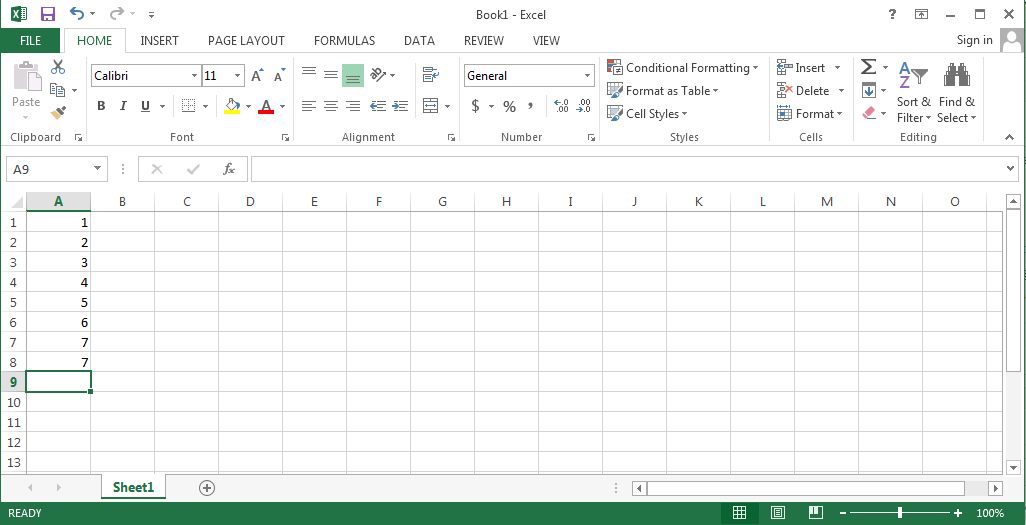Make sure to enter all the values in column A.

Step #3: Now, in this final step of finding the mean. If you are aware of using the formula, then you can use the formula which is specified in the next statements. You are now required to click on the cell B1. Once you are in the B1 cell, then you now need to type the following formula: “=AVERAGE(A: A)”.

Alternatively, you can follow this method. For this, you will have to select the data entries first and then click on the fx option and then follow as the pictures say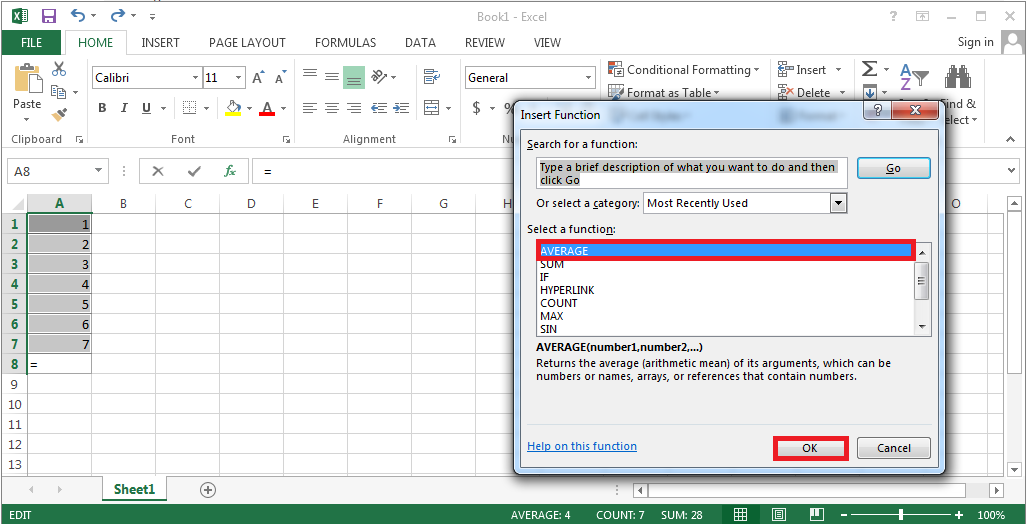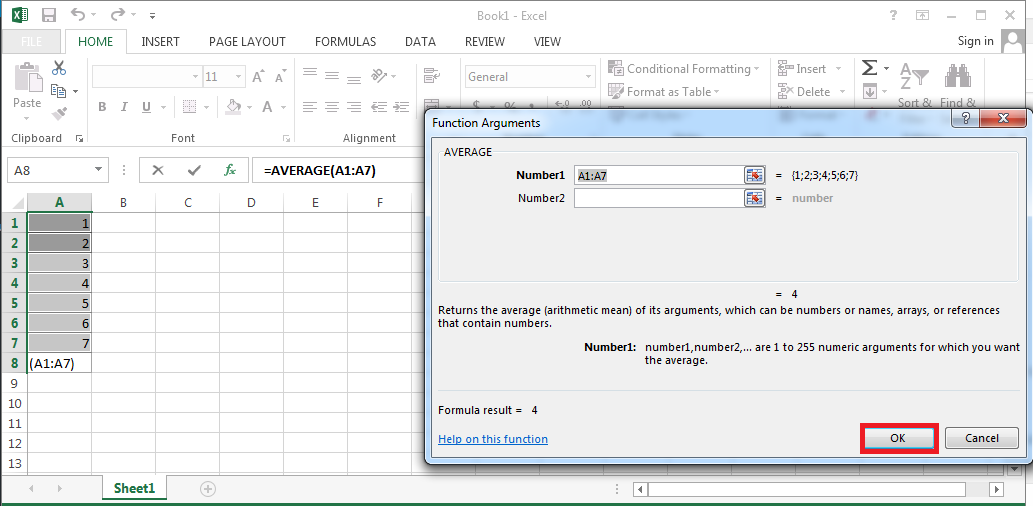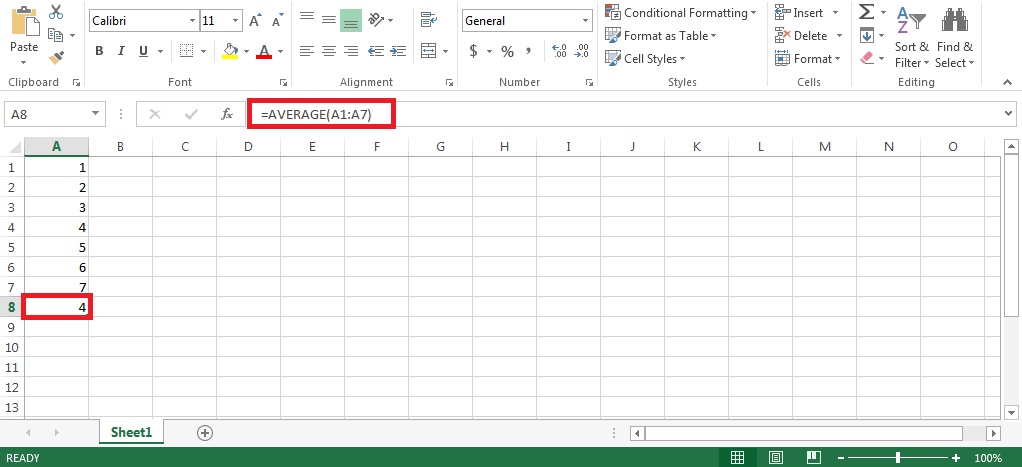Once you are done entering the formula then all that you need to do is to hit enter and this step of yours will display the average or mean of the set of values which you have entered.

Additionally, if you want to calculate the mean and median of the set of values then you can also do the same by entering the corresponding formula. To calculate the median, you are required to enter “=MEDIAN(A:A)” without quotes at the B2 cell. After this, you only required to hit enter and the median will be displayed instantly.

To calculate Mode, you are required to enter the following formula: “=MODE.MULT(A:A)” in B3 cell. After this, you only need to hit enter and this way Mode will be displayed. Do math on MS Excel for faster and accurate results. Here is how to divide in Excel.

### Method 3:

Alternatively, if you do not want to do all the calculations yourself then you can consider going for some online services.
For that, you will have to make a search on your favorite search engine with the terms akin to “online mean calculator”, “how to find mean online?” or “mean calculator online”.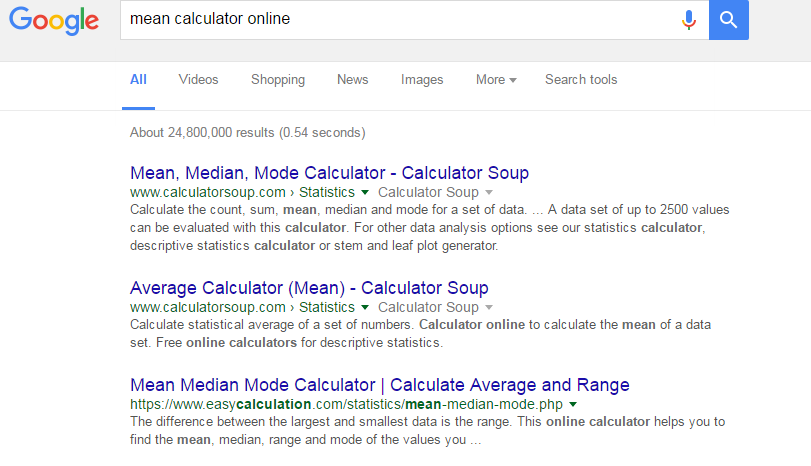For the sake of convenience, we will show you how to calculate mean by using such online platforms. Let’s say, for instance, we took the platform named as “Calculatorsoup”. So, let’s get started with the same.

Step #1: As the very first step of this method, you are required to visit the following “www.calculatorsoup.com” or you can directly visit this link for the mean calculator. Once you have reached there then you can now move on to our next steps.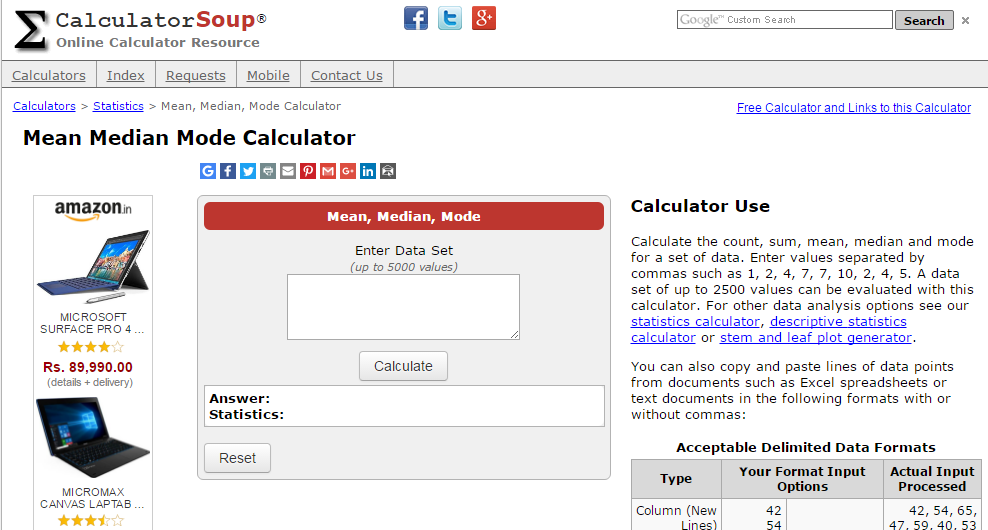Step #2: Now, you are required to enter the data values in the text field above which Enter Data Set is written.You can add up to 5000 values at a time.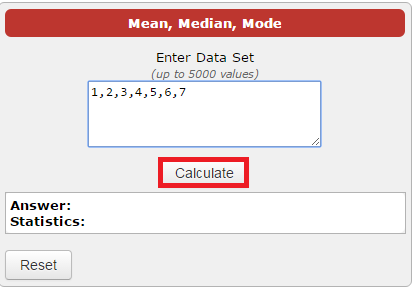You will have to enter the data set of values separated by commas. Once you are done entering the values then all that you require doing is to click on “Calculate”.

Step #3: Now, since you have clicked on the “Calculate” button so, you will have the result in front of you as shown below. Additionally, you will also have the median and mode along with mean. An added bonus for you as well.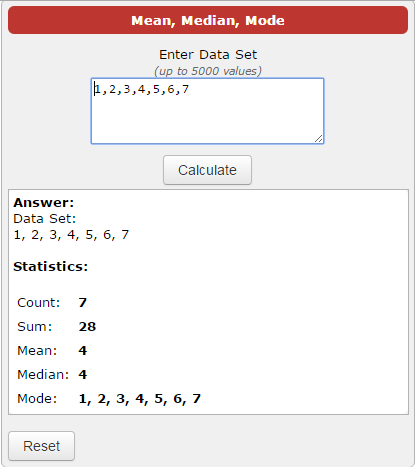So, this was all about “How to find mean in maths?“. We hope that after going through this article, you will be able to calculate mean easily.

How do you find the mean?  If you are aware of any other methods, then do let us know through the comments section below. Your addition is highly appreciated. If you have big number for addition, here is how to Add Numbers in Excel.

If you liked this article and found it noteworthy then do not forget to share it with your peers.

Thank you for crossing by and giving it a read.

Stay tuned and keep visiting.##### arun singh

Arun Singh is a experienced server management geek and he has more than 8 years of experienced handling hosting server and he do sometimes share his knowledge in digital marketing. He currently with private company in Mumbai, India and he handles server management at BloggersIdeas.com.